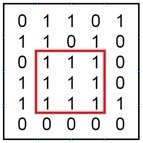# Maximum size square submatrix with all 1s

When a binary matrix is given, our task is to find a square matrix whose all elements are 1.

For this problem, we will make an auxiliary size matrix, whose order is the same as the given matrix. This size matrix will help to represent, in each entry Size[i, j], is the size of a square matrix with all 1s. From that size matrix, we will get the maximum number to get the size of the biggest square matrix.

## Input and Output

Input:
The binary matrix.
0 1 1 0 1
1 1 0 1 0
0 1 1 1 0
1 1 1 1 1
0 0 0 0 0

Output:
The largest submatrix with all 1’s.## Algorithm

subMatWithOne(given matrix)

Input − The main matrix.

Output − Display the square matrix with all 1, which one is the largest.

Begin
define subMat whose order is same as given matrix
copy first row and first column of given matrix to subMat

for all row i (1 to n), do
for all column j (1 to n), do
if matrix[i, j] = 1, then
subMat[i, j] := 1 + minimum of subMat[i, j-1]
and subMat[i-1, j-1]
else
subMat[i, j] := 0
done
done

maxSize := subMat[0, 0], iMax := 0 and jMax := 0
for all row i and column j, do
if maxSize < subMat[i, j], then
maxSize := subMat[i, j]
iMax := i, jMax := j
done

print sub matrix from row = iMax to (iMax - maxSize), and column jMax to (jMax - maxSize)
End

## Example

#include<iostream>
#define ROW 6
#define COL 5
using namespace std;

int matrix[ROW][COL] =  {
{0, 1, 1, 0, 1},
{1, 1, 0, 1, 0},
{0, 1, 1, 1, 0},
{1, 1, 1, 1, 0},
{1, 1, 1, 1, 1},
{0, 0, 0, 0, 0}
};

int min(int a, int b, int c) {
return ((a<b?a:b))?((a<c)?a:c):((b<c)?b:c);
}

void subMatWithOne() {
int subMat[ROW][COL];
int maxSize, iMax, jMax;

for(int i = 0; i < ROW; i++)    //copy first row of matrix to sub matrix
subMat[i] = matrix[i];

for(int j = 0; j < COL; j++)    //copy first column of matrix to sub matrix
subMat[j] = matrix[j];

for(int i = 1; i < ROW; i++) {
for(int j = 1; j < COL; j++) {
if(matrix[i][j] == 1)    //find minimum of left, top and diagonal element + 1
subMat[i][j] = min(subMat[i][j-1], subMat[i-1][j], subMat[i-1][j-1]) + 1;
else
subMat[i][j] = 0;    //if item is 0, put only 0
}
}

maxSize = subMat; iMax = 0; jMax = 0;
for(int i = 0; i < ROW; i++) {    //find the order of sub square matrix

for(int j = 0; j < COL; j++) {
if(maxSize < subMat[i][j]) {

maxSize = subMat[i][j];
iMax = i;
jMax = j;
}
}
}

cout << "Subsquare matrix: "<<endl;
for(int i = iMax; i > iMax - maxSize; i--) {    //print the submatrix using max size
for(int j = jMax; j > jMax - maxSize; j--) {
cout << matrix[i][j]<<" ";
}
cout << endl;
}
}

int main() {
subMatWithOne();
} 

## Output

Subsquare matrix:
1 1 1
1 1 1
1 1 1